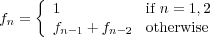# PreLab 02

The Basics
Due in class on Wednesday, September 6.

In this prelab you will familiarize yourself with some of the mathematical and design questions underlying Lab 02 by working on the first three steps of theprogramming process that we described in Lab 1(describing and understanding the problem and designing an algorithm). There are 5 exercises which appear in boxes below. Please type or write legibly your solutions and hand in a paper copy in class on Wednesday.

## Part 0 - Loop Practice

We will talk about loops in class on Wednesday. Meanwhile, they are discussed in my notes Secction 3.3 (and sections 3.4 and 3.5, but FOR-loops are all you need for this lab). For this prelab you don't need to write any code, just read some. What will these two programs print? Note that you can type them into a Python system to find the answer; make sure you understand why the code gives the output it does.

1.
```   for i in range(1,4) :
for j in range(1, 3) :
print(j)
print(i)
```

2.
```   for i in range(1, 5) :
for j in range(0, i) :
print("*", end="")
print("!")

If you don't understand that end="" in the print statement see p. 36 of the  text or section 2.6 of the Notes.```

## Part 1 - Loopin'

In this portion of the prelab you'll start thinking about how computers can handle repetition.

### Fibonacci

The Fibonacci numbers are a sequence of integers defined by the following recurrence relationIn other words, each Fibonacci number is the sum of the previous two.

Describe the Problem:
The problem you will solve on your lab is as follows.
input: get a positive integer n from the user.
goal: compute and output the nth Fibonacci number.

Understand the Problem:
For example, the first six Fibonacci numbers are 1, 1, 2, 3, 5, 8.

3. Compute the next eight Fibonacci numbers.

Hint: you should end with 377.

## Part 2 - Patterns, Patterns Everywhere

In the next batch of problems, we're going to be looking for patterns in sequences of figures. For each problem, a few figures are given, each of which made out of ASCII characters and is associated with an index (a number).

Describe the Problem:
The problem you will solve on your lab is as follows.
input: get a positive integer n that represents the index of your pattern.
output: print the nth pattern to the console, for whichever pattern you are currently on.

Understand the Problem:
For example, below are some values of n and their associated figures for Pattern A.

### Pattern A

Figure 3:

```    1 2 3
1 2 3
1 2 3

```
Figure 4:
```    1 2 3 4
1 2 3 4
1 2 3 4
1 2 3 4```

Figure 5:
```    1 2 3 4 5
1 2 3 4 5
1 2 3 4 5
1 2 3 4 5
1 2 3 4 5
```

You can probably see what's happening; figure 3 consists of 3 rows, each of which includes all numbers from 1 to 3. You can infer that figure 2 would look like

```    1 2
1 2
```
and similarly, figure 6 would look like
```    1 2 3 4 5 6
1 2 3 4 5 6
1 2 3 4 5 6
1 2 3 4 5 6
1 2 3 4 5 6
1 2 3 4 5 6
```

Line 1 of figure 6 is all numbers from 1 to 6. Line 2 of figure 6 is also all numbers from 1 to 6. In general, line i of figure 6 is all numbers from 1 to 6. Even more generally, we can give the following characterization of Pattern A:

Line i of figure n consists of all numbers from 1 to n.

Design an Algorithm:
In order to design an algorithm for this problem, we need to formulate a precise description of what the current pattern is. In particular, an algorithm to output pattern A is as follows:

• Get the index n from the user.
• For each i from 1 to n
• print out line i of figure n, which consists of all numbers from 1 to n

Below we will give you some different patterns. Your task is to (1) show that you understand each pattern, by giving the next pattern in the sequence, and (2) design an algorithm for the problem, by defining what an arbitrary line i of figure n would contain. Note that in the example above, the description of line i in figure n did not depend on i. In the following patterns, the description of a line may depend on i, n, or both.

### Pattern B

Figure 3:

```    1 1 1
2 2 2
3 3 3

```
Figure 4:
```    1 1 1 1
2 2 2 2
3 3 3 3
4 4 4 4

```
Figure 5:
```    1 1 1 1 1
2 2 2 2 2
3 3 3 3 3
4 4 4 4 4
5 5 5 5 5
```
4. Describe line i of figure n in Pattern B, i.e. "Line i of figure n consists of ....".

### Pattern C

Figure 3:

```    1 2 3
2 3
3

```
Figure 4:
```    1 2 3 4
2 3 4
3 4
4

```
Figure 5:
```    1 2 3 4 5
2 3 4 5
3 4 5
4 5
5
```

5. Describe line i of figure n in Pattern C.

## Honor Code

If you followed the Honor Code in this assignment, write the following sentence attesting to the fact at the top of your prelab:

I affirm that I have adhered to the Honor Code in this assignment.